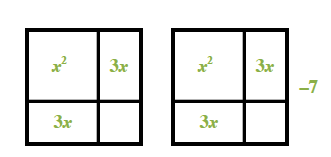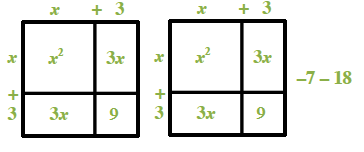### Home > INT2 > Chapter 10 > Lesson 10.1.1 > Problem10-8

10-8.

Complete the square to write $y = 2x^2 + 12x - 7$ in graphing form. Then determine the vertex and the intercepts of the parabola.

Make two perfect squares. 2 generic rectangles, each with interior labeled as follows: top left, x squared, top right & bottom left, each labeled 3, x. Bottom right blank. To the right of the second rectangle is the label, negative 7.

Added to the rectangles, top side & left side, on each, labeled, x, + 3. Interior bottom right each labeled, 9. After the negative 7, is the label negative 18.

Graphing form:
$y=2(x+3)^2-25$
Vertex: $(-3, -25)$ $y$-intercept: $(0, -7)$